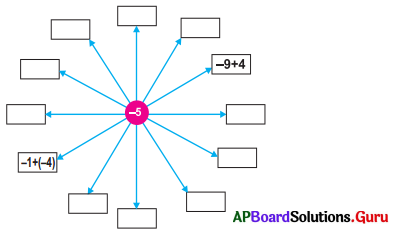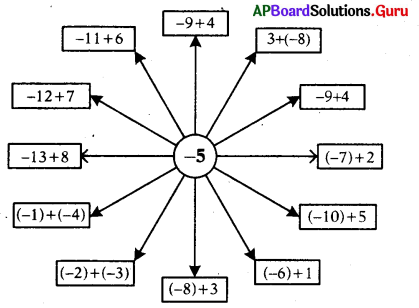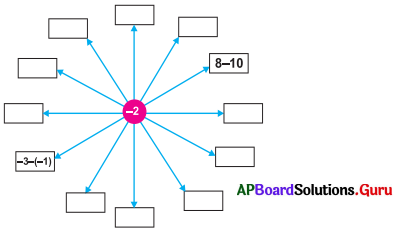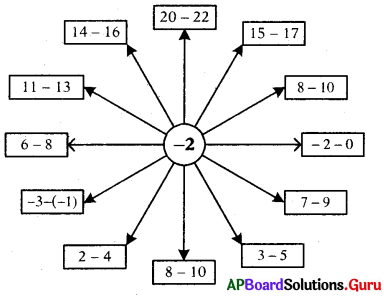# AP Board 7th Class Maths Solutions Chapter 1 Integers Review Exercise

SCERT AP 7th Class Maths Solutions Pdf Chapter 1 Integers Review Exercise Questions and Answers.

## AP State Syllabus 7th Class Maths Solutions 1st Lesson Integers Review Exercise

Question 1.
Represent the following statements with a suitable integer.
(i) Sneha deposited ₹ 2000 in her savings account.
+ 2000 Rupees.(ii) A submarine is in the depth of 350 feet in the sea level.
– 350 feet.

(iii) The height of Mount Everest is 8848 m J 3 above the sea level.
+ 8848 m.

(iv) Temperature of 14 degrees below 0° C.
– 14°C.

Question 2.
Fill the missing integers on the number line.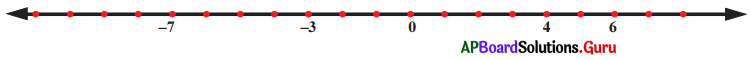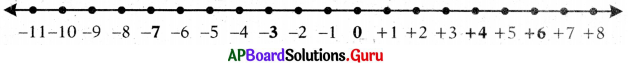Question 3.
Represent the following additions and subtractions on number line.
(i) 7 + 4
(ii) – 8 + 3
(iii) 9 – 11
(iv) 13 – 5

Question 4.
Write the following integers in descending order and ascending order.
(i) -9, -1, 0, -10, -6
Ascending order: -10, -9, -6,- 1, 0
Descending order: 0, -1, -6, -9,-10(ii) -6, 6, -9, 5, 10, -3
Ascending order: -9, -6, -3, 5, 6, 10
Descending order: 10, 6, 5, -3, -6, -9

(iii) 15, -20, -35, 0, 2
Ascending order: -35, -20, -15, 0, 2
Descending order: 2, 0, -15, -20, -35

Question 5.
Calculate the following.
(i) – 2 + 3
– 2 + 3 = +1

(ii) – 6 + (- 2)
– 6 + (- 2) = – 6 – 2 = – 8

(iii) 8 – (- 6)
8 – (-6) = 8 + 6 = + 14

(iv) – 9 + 4
– 9 + 4 = – 5

(v) – 23 – (- 30)
– 23 – (- 30) = – 23 + 30 = + 7

(vi) 50 – 153
50 – 153 = + 50 – 153 = – 103

(vii) 71 + (- 10) – 8
71 + (-10) – 8 = + 71 – 10 – 8
= + 71 – 18 = + 53(viii) – 30 + 58 – 38
-30 + 58 – 38 = – 30 – 38 + 58
= – 68 + 58 = – 10

Question 6.
The temperature in Siachen at 5 a.m. was 10 degrees below 0°C. Six hours later, it had increased to 14°C. What is the temperature at 11 a.m. ?Temperature at 5 a.m. = 10 degrees below 0°C = – 10°C
Six hours later increased temperature = + 14°C
Temperature at 11 a.m. = -10°C + 14°C
= +4°C
∴ Temperature at 11 a.m. in Siachen is + 4°C.

Question 7.
A fish was at 16 feet below the surface of sea and went down another 17 feet. What is its position now from the surface of sea?Place of the fish in the beginning = 16 feet below surface = -16 feet
Place of the fish at last from the beginning = – 17 feet
∴ Position of fish now = – 16 + (-17)
= – 16 – 17
= – 33 feetQuestion 8.
A green grocer had a profit of ₹ 250 on Monday, a loss of ₹ 120 on Tuesday and loss of ₹ 180 on Wednesday. Find total profit or loss after 3 days.
Profit on Monday = + ₹ 250
Loss on Tuesday = – ₹ 120
Loss on Wednesday = – ₹ 180
Total loss two days = – 120 + (- 180)
= – 120 – 180 = – 300
Loss is greater than profit. So, he will get loss.
∴ Total loss on three days = + 250 + (-300)
= + 250 – 300
= – 50
= – ₹ 50

Question 9.
Fill the empty boxes in first diagram by doing addition of two integers and in second diagram by doing subtraction of two integers.
(i)